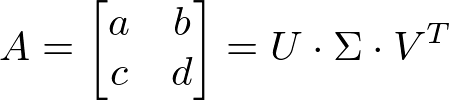# How to derive the formula of the curvature of a curve# General Leibniz rule Explained# Visualizing the inverse theorem# Maxima cheat sheet# A geometric interpretation of the cross product# Tensor basic definition# Avoid SVD to compute optimal rotation between point setsDraft note

# Polar decomposition of a 3x3 matrixLeaving [C++ code ] to decompose a 3x3 matrix into rotation and scale/shear with polar decomposition.Cheat sheet to differentiate expressions with the $\nabla$ operator to compute gradients of various functions.

# Singular value decomposition of a 2x2 matrix (C++ code)Dropping some [code here] to do a singular value decomposition (SVD) of a 2 by 2 real matrix.

# C code for 4x4 matrix inversion

Just leaving some code here to invert either column or row major 4x4 matrices.

• 1